2023-05-1000

## 引言

• $O(n^2)$：冒泡排序，插入排序，选择排序。

• $O(nlogn)$：快速排序，归并排序。

• $O(n)$：桶排序，计数排序，基数排序。

• 排序算法的执行效率 - 时间复杂度

• 排序算法的内存消耗 - 原地排序算法/非原地排序算法

• 排序算法的稳定性 - 稳定排序算法/不稳定排序算法

## 冒泡算法

### 算法图解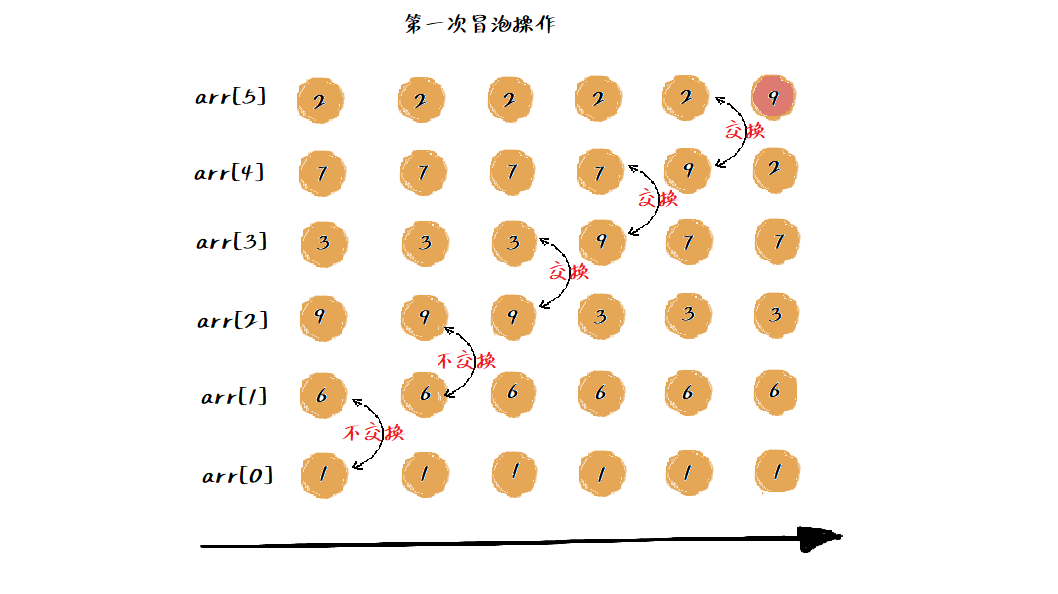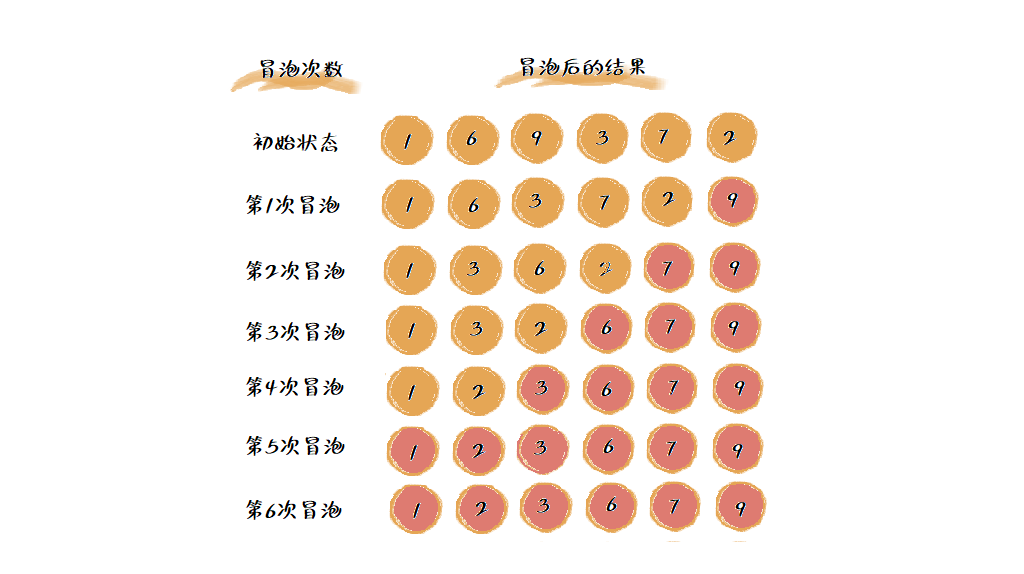csharppublic void BubbleSort(int[] arr)
{
if (arr == null || arr.Length == 0)
return;

var length = arr.Length;
//提前结束排序的标志
bool flag = false;

for (int i = 0; i < length; i++)
{
for (int j = 0; j < length - i - 1; j++)
{
if (arr[j] > arr[j + 1])
{
//交换元素
int temp = arr[j];
arr[j] = arr[j + 1];
arr[j + 1] = temp;

flag = true;
}
}
//如果没有数据交换，则提前退出
if (!flag)
break;
}
}



### 算法分析

1. 稳定性

冒泡排序只涉及到相邻数据交换，只需要常量级的临时空间，空间复杂度为$O(1)$，是一个原地排序算法。

2. 内存消耗

冒泡排序中，只有交换才可以改变两个元素的前后顺序，为了保证冒泡排序的稳定性，在冒泡过程中，我们对两个大小相等的相邻的元素不做交换，这就保证了相同大小的元素顺序在排序后不会改变。因此冒泡排序是一个稳定排序算法。

3. 时间复杂度

最好的情况下，要排序的数据已经是有序的了，我们只需要进行一次冒泡操作，所以最好时间复杂度为$O(1)$

最坏的情况下，要排序的数据是倒序排列的，则需要 $n$ 次冒泡操作，因此最坏时间复杂度为$O(n^2)$

对于平均时间复杂度，假设有 $n$ 个数据的集合，有 $n!$ 种不同的排列方式，所以很难直接计算时间复杂度。

• 有序度

有序度是指数组中具有有序关系的元素对的个数，如果用数学式表达出来，就是：

$a[i] \leq a[j], \quad i
• 逆序度

逆序度则跟有序度定义相反，是指数组中逆序元素对的个数（假设从小到大为有序） ，如果用数学式表达出来，就是：

$a[i] > a[j], \quad i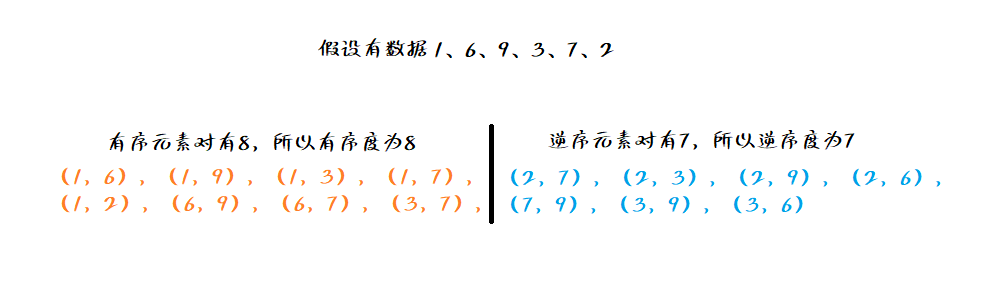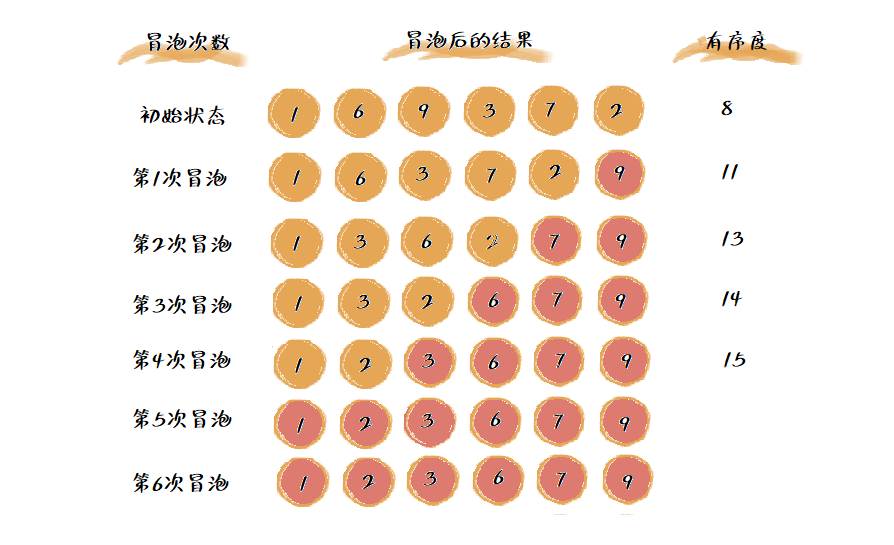可以看到冒泡排序只有比较和交换两个操作，因为交换只会交换相邻两个元素，所以每一次交换，有序度就增加1。无论冒泡算法如何改进，它的总交换数是固定的，这个数也是逆序度，所以上图排序过程中的满排序度是15（$\frac{6(6-1)}{2}$），初始有序度为8，所以上述排序过程共进行了7次交换操作。

## 插入排序

### 算法图解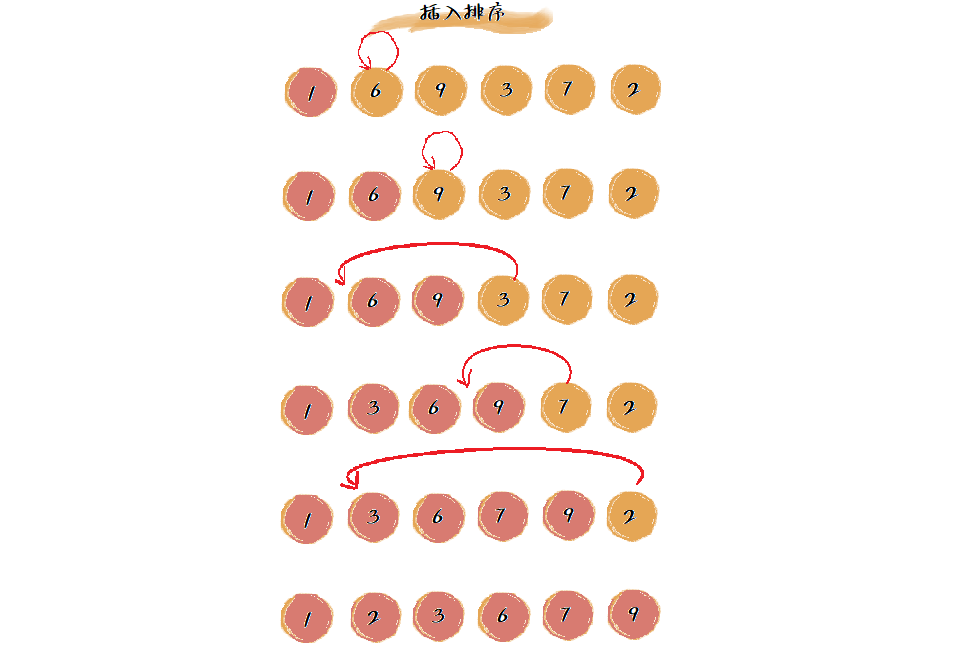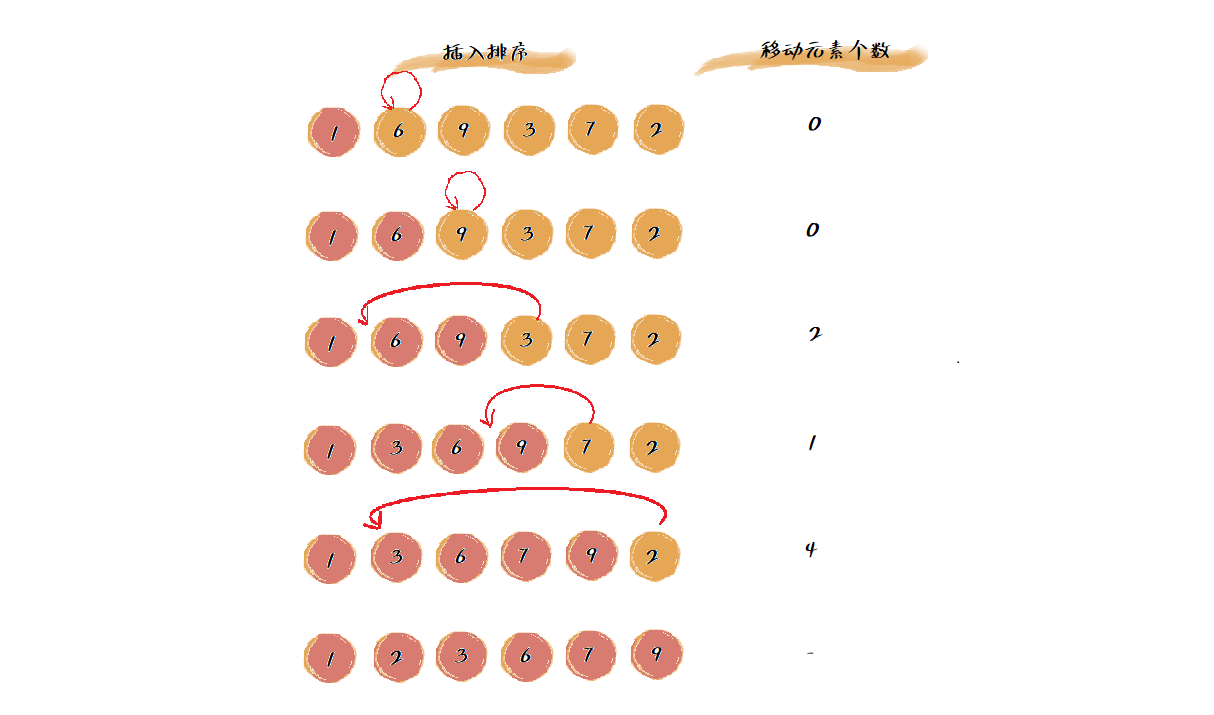与冒泡排序算法一样，我们再来回答下面 3 个问题。 通过原理介绍和代码实现，我们可以很明显地看出，插入排序的运行过程并不需要额外的存储空间，因此，它是原地排序算法。同时，它的空间复杂度也是 $O(1)$

csharppublic static void InsertionSort(int[] arr)
{
if (arr == null || arr.Length == 0) return;

var length = arr.Length;

//从第二个元素开始比较（未排序区间）
for (int i = 1; i < length; i++)
{
var val = arr[i];

//遍历已排序区间
for (int j = i - 1; j >= 0; j--)
{
//按从小到大排序，所以如果目标元素比已排序元素小，则已排序区间往后移动一位
if (arr[j] > val)
{
arr[j + 1] = arr[j];
}
else
{
arr[j + 1] = val;
break;
}
}
}
}



## 选择排序

### 算法图解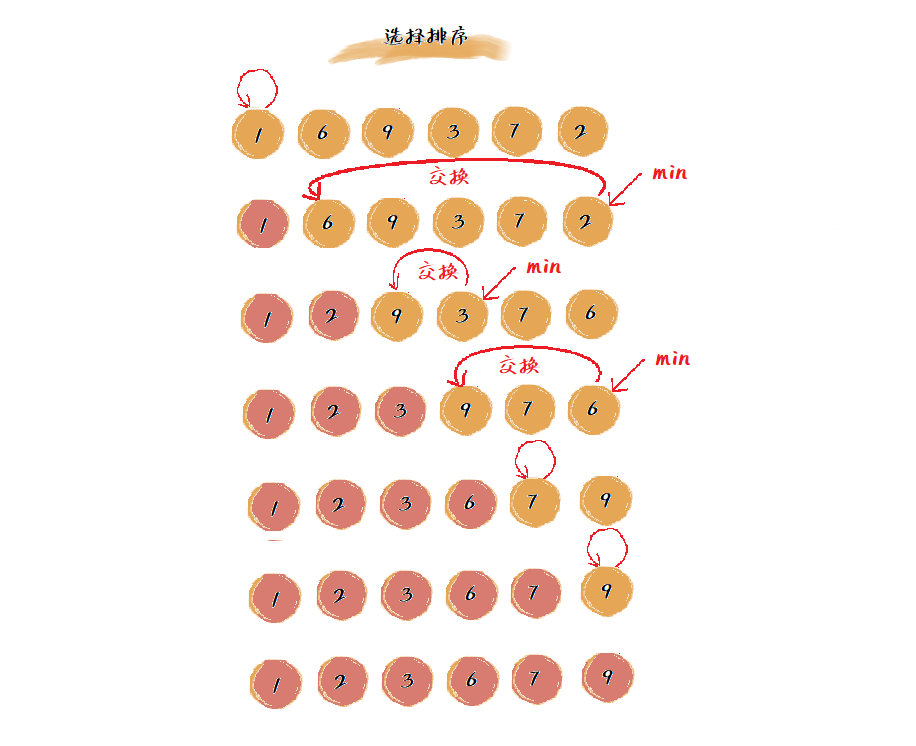csharppublic static void SelectionSort(int[] arr)
{
if (arr == null || arr.Length == 0) return;

var length = arr.Length;
//只需循环length - 1次
for (int i = 0; i < length - 1; i++)
{
int minPos = i;
//找出最小值的索引
for (int j = i; j < length; j++)
{
if (arr[j] < arr[minPos])
{
minPos = j;
}
}
//交换元素
int temp = arr[i];
arr[i] = arr[minPos];
arr[minPos] = temp;
}
}


### 算法分析

 数据结构与算法之美 / 王争 著. --北京：人民邮电出版社，2021.6